Courses

# Synchronous Machine Armature Windings - 1 Notes | EduRev

## Electrical Engineering (EE) : Synchronous Machine Armature Windings - 1 Notes | EduRev

The document Synchronous Machine Armature Windings - 1 Notes | EduRev is a part of the Electrical Engineering (EE) Course Electrical Machines.
All you need of Electrical Engineering (EE) at this link: Electrical Engineering (EE)

Winding Types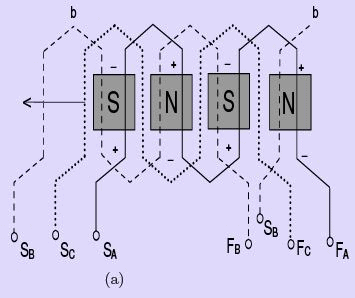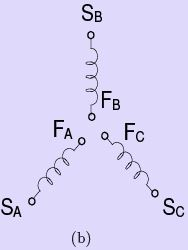Figure 8: Concentrated three-phase,half-coil wave winding with one slot per phase(one coil side per slot and instantaneous polarity and phase relation of coils)

A three phase winding, in extremely simpliﬁed form, is shown in Fig. 8. The start and ﬁnish of all the coils in phase A are designated, respectively, as SA and FA. Phase A is shown as a solid line in the ﬁgure, phase B as a dashed line, and phase C as a dotted line. Note that each winding does not start and ﬁnish under the same pole. Further, note that the two coil sides of a given coil lie in identical magnetic conditions of opposite polarity.
This implies that when seen from the coil terminals, the emfs produced in the two coil sides add up. If we assume that the poles on the rotor are moving to the left as shown, then the relative motion of the armature conductors is to the right. This implies that identical magnetic conditions will be seen by conductors of phase A, followed by phase C, followed by phase B. The induced emfs in phases A,C and B may be said to produce a phase sequence of ACBACBA.The time interval between two phases to achieve identical magnetic conditions would depend on the relative speed of motion, and on the spatial seperation of the phases. In Fig 8, the phases are so laid out that each phase is seperated from another by 120 electrical degrees (360◦ being deﬁned by the distance to achieve identical magnetic conditions).

As the distance between two adjacent corresponding points on the poles is 180 electrical degrees, we can see that the distance between the coil side at the start of A and that at the start of C must be 120 electrical degrees. Thus, the leading pole tip of a unit north pole moving to the left in Fig. 8 will induce identical voltages in corresponding coil sides A, C, and B, respectively, 120 electrical degrees apart. Note that phase B lags phase A by 240 electrical degrees or leads phase A by 120 electrical degrees.Fig. 8(b) is a representation that is frequently used to depict the windings of the three phases and the phase relationship between them.

The winding depicted in Fig. 8 is an open winding since both ends of the windings have been brought out for suitable connections. It is a wave winding since it progresses from pole to pole. It is a concentrated winding because all the coils of one phase are concentrated in the same slot under one pole. It is a half-coil winding because there is only one-half of a coil (one coil side) in each slot. It is a full-pitch winding because the coil sides of one coil are 180◦ electrical degrees apart i.e., they lie under identical magnetic conditions, but of opposite polarity under adjacent poles.

Fig. 9, on the other hand shows the coils of a single phase,(A, in this case) distributed winding distributed over two slots under each pole.

Half-coil and whole-coil windings

Half-coil (also called single-layer) windings are sometimes used in small induction motor stators and in the rotors of small wound-rotor induction motors. A cross section of a half-coil, single-layer winding is shown in Fig. 9(c)(i). Like the dc dynamo armature windings, most commercial armatures for ac synchronous generators are of the full or wholecoil two-layer type, shown in cross section at the right in Fig. 9(c)(ii). The whole-coil, two-layer winding gets its name from the fact that there are two coil sides (one coil) per slot.
Fig. 9(a) shows a single-layer, half-coil lap windings;Fig. 9(b) shows a double-layer, full-coil lap winding. A cross section of a single layer (half-coil) winding is shown in Fig. 9(c)(i)

Chorded or fractional -pitch windings

Whereas most single-layer windings are full-pitch windings, the two-layer, whole-coil windings are generally designed on an armature as a chorded or fractional-pitch windings.
This common practice stems from the fact that the primary advantage of the whole-coil windings is that it permits the use of fractional-pitch coils in order to save copper. As will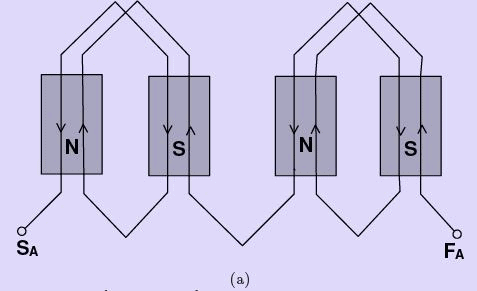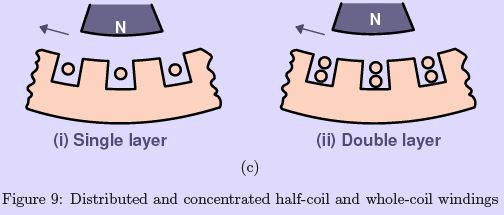be shown later, fractional-pitch windings, when used in ac synchronous and asynchronous generator armatures, in addition to saving copper, (1) reduce the MMF harmonics produced by the armature winding and (2) reduce the EMF harmonics induced in the windings, without reducing the magnitude of the fundamental EMF wave to a great extent. For the three reasons cited, fractional-pitch windings are almost universally used in ac synchronous generator armatures.

EMF of Fractional Pitch Windings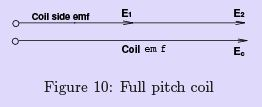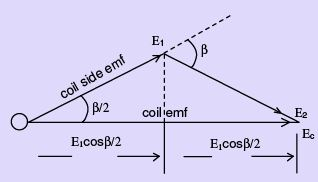Figure 11: Fractional-pitch coil - Coil EMF in terms of coil side EMFs for fractional-pitch coil

In the case of an ac generator using a full-pitch coil, such as that shown in Fig. 8, the two coil sides span a distance exactly equal to the pole pitch of 180 electrical degrees. As a result, the EMFs induced in a full-pitch coil are such that the coil side EMFs are in phase, as shown in Fig. 10. The total coil voltage Ec is 2E1 , if E1 is the emf induced in a coil-side.

In the case of the two-layer winding shown in Fig. 9(b), note that the coil span of single coil is less than the pole span of 180 electrical degrees. The EMF induced in each coil side is not in phase, and the resultant coil voltage Ec would be less than the arithmetic sum of the EMF of each coil side, or less than 2E1. It is obvious that 2E1 must be multiplied by a factor,kp, that is less than unity, to get the proper value for coil voltage E(or E= 2Ekp).
The pitch factor kis given by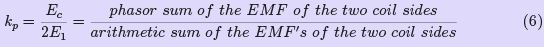The pitch factor may be quantiﬁed in terms of angles as follows. If we assume that the induced EMFs of two coils, E1 and E2 , are out of phase with respect to each other by some angle β as shown in Fig. 11, then the angle between E1 and the resultant coil voltage Ec is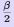.The resultant coil voltage Ec is from Eqn. 6 and Fig. 11.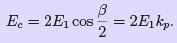(7)
and, therefore,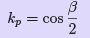(8)
The angle β is 180minus the number of electrical degrees spanned by the coil, for a shortpitched coil. For a full pitched coil, therefore, kp = 1 as β = 0.

Since β is the supplementary of the coil span, the pitch factor kp may also be expressed as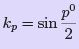(9)
where p0 is the span of the coil in electrical degrees.

It is sometimes convenient to speak of an armature coil span as having a fractional pitch expressed as a fraction e.g.,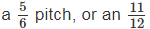pitch, etc. This fraction is infact the ratio of the number of slots spanned by a coil to the number of slots in a full pitch.
In such a case, the electrical degrees spanned,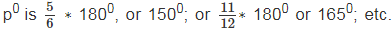The pitch factor kis still computed as in Eqn. 9. Over pitched coils are not normaly used in practice as there is an increased requirement of copper wire without any additional advantage.

Relation between Electrical and Mechanical Degrees of Rotation

As stated earlier there are 180 electrical degrees between the centres of two adjacent north and south poles. Since 360 electrical degrees represents a full cycle of sinusoidal EMF,
we are interested in determining how many sinusoidal cycles are generated in one complete mechanical rotation, i.e., 360 mechanical degrees for a machine having P poles. The number of electrical degrees as a function of degrees of mechanical rotation is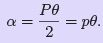(10)
where P is the number of poles (always an even integer), p is the number of pole-pairs, and θ is the number of mechanical degrees of rotation.

Thus, a two-pole machine generates one cycle of sinusoid; a four-pole machine generates two cycles and so on, in one full revolution of the armature.

Distributed windings and distribution (or Belt) factor

The windings shown in Fig. 8 and Fig. 9(b) are called concentrated windings because all the coil sides of a given phase are concentrated in a single slot under a given pole. For Fig. 8., in determining the induced ac voltage per phase, it would be necessary only to multiply the voltage induced in any given coil by the number of series-connected coils in each phase. This is true for the winding shown in Fig. 8 because the conductors of each coil, respectively, lie in the same position with respect to the N and S poles as other series coils in the same phase. Since these individual coil voltages are induced in phase with each other, they may be added arithmetically. In otherwords, the induced emf per phase is the product of the emf in one coil and the number of series connected coils in that phase.

Concentrated windings in which all conductors of a given phase per pole are concentrated in a single slot, are not commercially used because they have the following disadvantages,

1. They fail to use the entire inner periphery of the stator iron eﬃciently.

2. They make it necessary to use extremely deep slots where the windings are concentrated. This causes an increase in the mmf required to setup the airgap ﬂux.

3. The eﬀect of the second disadvantage is to also increase the armature leakage ﬂux and the armature reactance.

4. They result in low copper-to-iron ratios by not using the armature iron completely.

5. They fail to reduce harmonics as eﬀectively as distributed windings.

For the ﬁve reasons just given, it is more advantageous to distribute the armature winding, using more slots and a uniform spacing between slots, than to concentrate the windings in a few deep slots.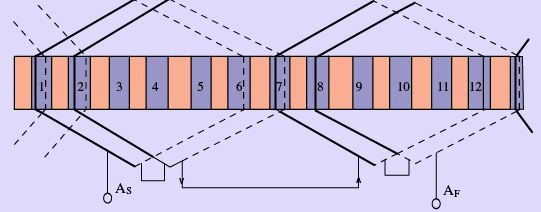Figure 12: Lap winding

When the slots are distributed around the armature uniformly, the winding that is inserted is called a distributed winding. A distributed lap winding is shown in Fig. 12.
Note that two coils in phase belt A are displaced by one slot angle (the angular displacement between two successive slots) with respect to each other. The induced voltages of each of these coils will be displaced by the same degree to which the slots have been distributed, and the total voltage induced in any phase will be the phasor sum of the individual coil voltages.

For an armature winding having four coils distributed over say, 2/3 rd of a pole-pitch, in four slots, the four individual coil side voltages are represented by phasors in Fig. 13 as displaced by some angle α, the number of electrical degrees between adjacent slots, known as slot angle. It is 300 for the case of 4 slots per phase belt. Voltages Ec1, Ec2, etc., are the individual coil voltages, and n is the number of coils in a given phase belt, in general.

For a machine using n slots for a phase belt, the belt or distribution factor kd by which the arithmetic sum of the individual coil voltages must be multiplied in order to yield the phasor sum is determined by the following method,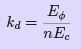(11)
where all terms are previously deﬁned

As in the case of Eqn. 12., the computation of kd in terms of voltages (either theo-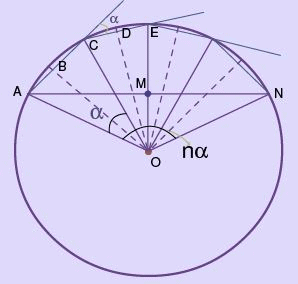Figure 13: Determination of distribution factor

retical or actual) is impractical. The construction of Fig. 13 in which perpendiculars have been drawn to the center of each of the individual coil voltage phasors to a common center of radius ’r’ (using dashed lines) serves to indicate that α/2 is the angle BOA. Coil side voltage AB equals OA sin α/2, and coil voltage represented by chord AC equals 2OA sin α/2. For n coils in series per phase, chord AN , is also 2OA sin nα/2, and the distribution or belt factor kd is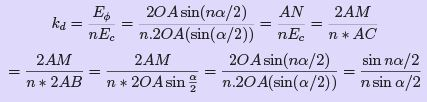where n is the number of slots per pole per phase (s.p.p) α is the number of electrical degrees between adjacent slots i.e. slot angle It should be noted from Eqn. 12. that the distribution factor kd for any ﬁxed or given number of phases is a sole function of the number of distributed slots under a given pole.
As the distribution of coils (slots/pole) increases, the distribution factor kd decreases. It is not aﬀected by the type of winding, lap or wave, or by the number of turns per coil, etc.

Generated EMF in a Synchronous Generator

It is now possible to derive the computed or expected EMF per phase generated in a synchronous generator. Let us assume that this generator has an armature winding consisting of a total number of full pitched concentrated coils C, each coil having a given number of turns Nc. Then the total number of turns in any given phase of an m-phase generator armature is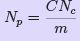(12)
But Faraday’s law Sec. ?? states that the average voltage induced in a single turn of two coil sides is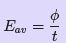(13)
The voltage induced in one conductor is 2φ/(1/s) = 2φs, where s=speed of rotation in r.p.s, for a 2 pole generator. Furthermore, when a coil consisting of Nc turns rotates in a uniform magnetic ﬁeld, at a uniform speed, the average voltage induced in an armature coil is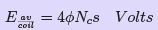(14)

where φ is the number of lines of ﬂux (in Webers) per pole, Nc is number of turns per coil, s is the relative speed in revolutions/second (rps) between the coil of Nc turns and the magnetic ﬁeld φ.
A speed s of 1 rps will produce a frequency f of 1 Hz. Since f is directly proportional and equivalent to s, (for a 2-pole generator) replacing the latter in Eqn. 14, for all the series turns in any phase,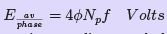(15)

However, in the preceding section we discovered that the voltage per phase is made more completely sinusoidal by intentional distribution of the armature winding. The eﬀective rms value of a sinusoidal ac voltage is 1.11 times the average value. The eﬀective ac voltage per phase is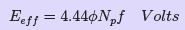(16)

But Eqn. 16 is still not representative of the eﬀective value of the phase voltage generated in an armature in which fractional-pitch coils and a distributed winding are employed.
Taking the pitch factor kp and the distribution factor kd into account, we may now write the equation for the eﬀective value of the voltage generated in each phase of an AC synchronous generator as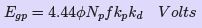(17)

Offer running on EduRev: Apply code STAYHOME200 to get INR 200 off on our premium plan EduRev Infinity!

61 docs|20 tests

,

,

,

,

,

,

,

,

,

,

,

,

,

,

,

,

,

,

,

,

,

;